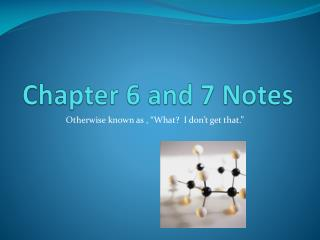# Chapter 6 and 7 Notes - PowerPoint PPT PresentationDownload PresentationChapter 6 and 7 Notes

Chapter 6 and 7 Notes
Download Presentation## Chapter 6 and 7 Notes

- - - - - - - - - - - - - - - - - - - - - - - - - - - E N D - - - - - - - - - - - - - - - - - - - - - - - - - - -
##### Presentation Transcript

1. Chapter 6 and 7 Notes Otherwise known as , “What? I don’t get that.”

2. Introduction to Bonding • Ionic Bonding • Transfer of electrons • Crystalline structure • Electrostatic attraction • Covalent Bonding • Sharing electrons • Polar or Non-polar • Hydrogen Bonding • Weak bond with Hydrogen

3. Compounds • Covalent bonds form molecular compounds • Lewis dot structure shows shared electrons and bond(s) formed – single, double, triple • Low melting points and boiling points • Polyatomic ions formed • Ionic bonds form ionic compounds • Lewis dot structure shows electron movement and ionic charges • High melting and boiling points

4. VSEPR Theory • Valence Shell, Electron Pair Repulsion • States that the repulsion between the sets of valence-level electrons surrounding an atom causes these sets to be oriented as far away as possible. • Molecular shapes as summarized on page 186 (6-5) • Shared electron pairs and atoms cause repulsion • Hybridization occurs between orbitals on different levels averaging out energy. • Intermolecular forces cause dipoles to occur which can lead to hydrogen bonding.

5. What is a Chemical Formula? • A chemical formula indicates the relative number of atoms of each type in a chemical compound. • Main Group elements form monatomic ions (p. 205) • Ions are named thusly: • Cation = element name Anion = element + ide • For example: Sodium Chloride • D-block elements and others form multiple ions

6. Binary Ionic Compounds • Formulas • Write the symbol for each ion side-by-side. • Cross over charges. • Check subscripts and divide by largest common factor. • Nomenclature • Stock = charge converted to Roman numeral for cation • Traditional = -ous is lesser charge –ic is greater charge

7. Oxyanions • Oxyanions = polyatomic ion that contains oxygen • If multiple ions the most common ends in –ate, the lesser ends in –ite. If more formed ite-1 = hypo, ate+1 = per. • Hypochlorite chlorite chlorate perchlorate

8. Molecular Compounds and Acids • Molecular • Prefix added to compound name (p. 212) • Mono not used at very beginning • Acid • Memorize chart on page 214 • ate goes to ic • ite goes to ous • Add hydro to beginning for element

9. Formula Mass and Molar Mass • Add the average atomic mass from the Periodic Table for each element times the number of each atom. • Percentage Composition is the percentage of each element in a compound. • (Mass of Element/Mass of Compound) x 100 • Empirical mass can be calculated using percentage of each element.